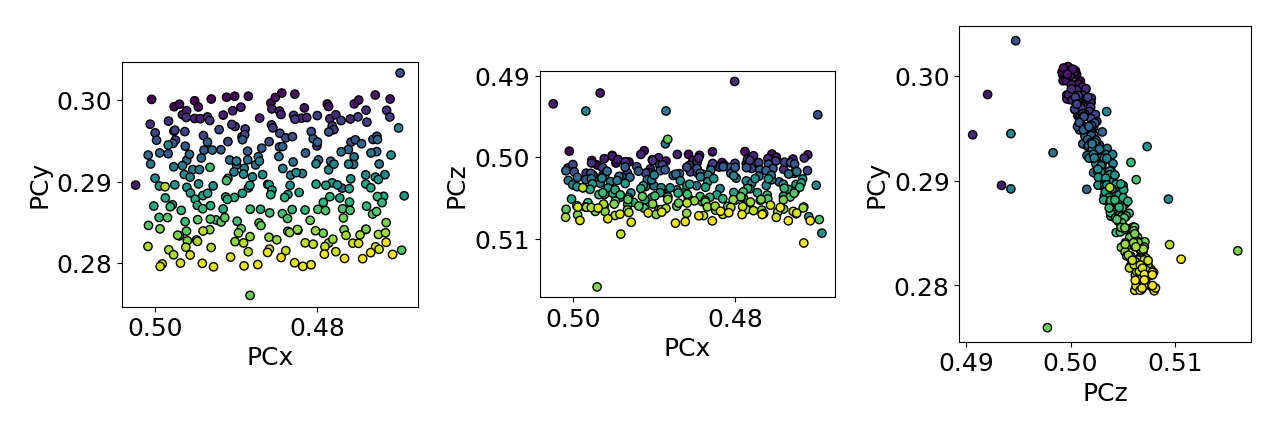# Estimate tilts about the detector x and z axis#

This example shows how to (robustly) estimate the tilts about the detector $$X_d$$ and $$Z_d$$ axes, which bring the sample plane normal into coincidence with the detector plane normal (but in the opposite direction) .

Estimates are found using estimate_xtilt_ztilt(), which fits a hyperplane to pc using singular value decomposition.

To test the estimations, we add some noise to realistic projection center (PC) values (PCx, PCy, PCz). The realistic PCs are extrapolated from a PC in the upper left corner of a map, assuming a nominal sample tilt of 70 degrees, a detector tilt of 0 degrees, a detector pixel size of 70 microns and a sample step size of 50 microns.True/estimated tilt about detector x [deg]: 20.00/20.66
True/estimated tilt about detector z [deg]: 0/1.65


import kikuchipy as kp
import matplotlib.pyplot as plt
import numpy as np

plt.rcParams["font.size"] = 18

# Create an initial detector with one PC assumed to be for the upper
# left corner of a map
det0 = kp.detectors.EBSDDetector(
shape=(480, 480),
pc=(0.5, 0.3, 0.5),
sample_tilt=70,
tilt=0,
px_size=70,
)

# Extrapolate a map of PCs
nav_shape = (15, 20)
nav_size = np.prod(nav_shape)
det = det0.extrapolate_pc(
pc_indices=[0, 0],
step_sizes=(50, 50),
)

# Add +/- 0.0025 as random noise
dev_noise = 0.001
rng = np.random.default_rng()
det.pcx += rng.uniform(-dev_noise, dev_noise, nav_size).reshape(nav_shape)
det.pcy += rng.uniform(-dev_noise, dev_noise, nav_size).reshape(nav_shape)
det.pcz += rng.uniform(-dev_noise, dev_noise, nav_size).reshape(nav_shape)

dev_outlier = 0.01
n_outliers = 20
outlier_idx1d = rng.choice(nav_size, n_outliers, replace=False)
is_outlier = np.zeros(nav_size, dtype=bool)
is_outlier[outlier_idx1d] = True
det.pcx[outlier_idx2d] += rng.uniform(-dev_outlier, dev_outlier, n_outliers)
det.pcy[outlier_idx2d] += rng.uniform(-dev_outlier, dev_outlier, n_outliers)
det.pcz[outlier_idx2d] += rng.uniform(-dev_outlier, dev_outlier, n_outliers)

# Plot PC values
det.plot_pc("scatter")

# Robust estimation by detecting outliers
xtilt, ztilt = det.estimate_xtilt_ztilt(degrees=True)

# Print true tilt and estimated tilt
true_xtilt = 90 - det.sample_tilt + det.tilt
print(f"True/estimated tilt about detector x [deg]: {true_xtilt:.2f}/{xtilt:.2f}")
print(f"True/estimated tilt about detector z [deg]: {0}/{ztilt:.2f}")


Total running time of the script: ( 0 minutes 0.797 seconds)

Estimated memory usage: 9 MB

Gallery generated by Sphinx-Gallery